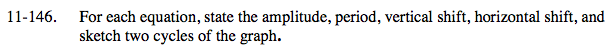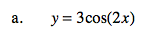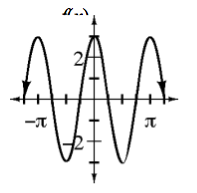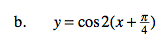### Home > A2C > Chapter Ch11 > Lesson 11.3.2 > Problem11-146

11-146.The functions have the form of
y = a cos(b(xh)) + k.Use 5 points to sketch one cycle and then repeat the pattern.
amplitude = 1
period = π

$\text{horizontal shift}=-\frac{\pi}{4}$